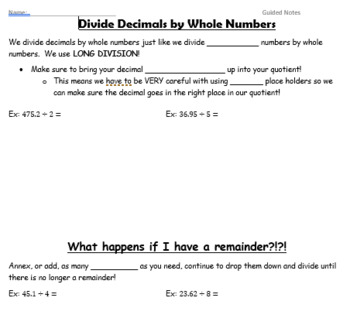# Decimal Operations Unit GUIDED NOTESSubject
Resource Type
File Type

Zip

(2 MB|12 pages)
Product Rating
Standards
5 Products in this Bundle
5 products
1. Guided Notes on adding and subtracting decimal numbers through thousandths. Answer key included.
2. Guided Notes on dividing decimals by decimals. Answer key included.
3. Guided Notes on dividing decimals by WHOLE numbers. Answer key included.
4. Guided Notes on multiplying decimal numbers by decimal numbers. Answer key included.
5. Guided Notes on multiplying decimals by WHOLE numbers. Answer key included.
• Bundle Description
• StandardsNEW

A unit of Guided Notes on Decimal Operations.

• Multiply Decimals by Whole Numbers
• Multiply Decimals by Decimals
• Divide Decimals by Whole Numbers
• Divide Decimals by Decimals

Fluently add, subtract, multiply, and divide multi-digit decimals using the standard algorithm for each operation.
Add, subtract, multiply, and divide decimals to hundredths, using concrete models or drawings and strategies based on place value, properties of operations, and/or the relationship between addition and subtraction; relate the strategy to a written method and explain the reasoning used.
Total Pages
12 pages
Included
Teaching Duration
N/A
Report this Resource to TpT
Reported resources will be reviewed by our team. Report this resource to let us know if this resource violates TpT’s content guidelines.